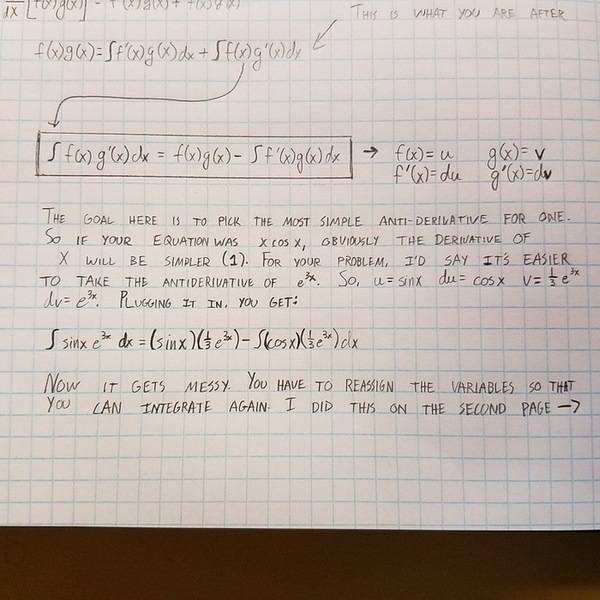# Integration by Parts Twice

## Homework Statement

Integrate e^3x sin x.

## Homework Equations

uv - Integral(v du)

## The Attempt at a Solution

I am trying to help somebody else with this problem, as I took Calculus a few years ago, but the end is really kicking my butt. I know I'm VERY close, but once I get to the second integration, I just get that u = cos x and du = -sin x. If I assign 1/3 e^3x to be dv, I just seem to get never ending equations in the integral that I can't remove. I know I'm very close, could someone give me a pointer in the right direction?Mark44
Mentor

## Homework Statement

Integrate e^3x sin x.

## Homework Equations

uv - Integral(v du)

## The Attempt at a Solution

I am trying to help somebody else with this problem, as I took Calculus a few years ago, but the end is really kicking my butt. I know I'm VERY close, but once I get to the second integration, I just get that u = cos x and du = -sin x. If I assign 1/3 e^3x to be dv, I just seem to get never ending equations in the integral that I can't remove. I know I'm very close, could someone give me a pointer in the right direction?You're on the right track. Do the same types of substitutions that you did the first time around, and you will have an equation involving the integral you're trying to evaluate. You can solve this equation algebraically.
In short, you will have something like this:
##\int f(x)dx = \text{stuff} + A \int f(x) dx##
##\Rightarrow (1 - A) \int f(x) dx = \text{stuff} + C##
You should be able to solve the last equation for the integral.